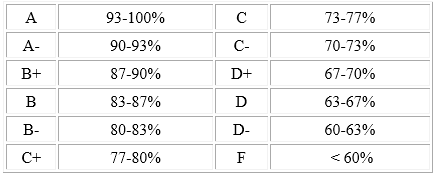# Midterm 1 and Current Course Grades in Finance 4366

I just uploaded the midterm 1 grades, along with attendance, quiz, problem set, and current Finance 4366 course grades to Canvas.

As stated in the course syllabus, final numeric course grades will be determined according to the following equation:

Final Course Numeric Grade =.10(Attendance and Participation) +.10(Quizzes) +.20(Problem Sets) + Max{.20(Midterm Exam 1) +.20(Midterm Exam 2) +.20(Final Exam),.20(Midterm Exam 1) +.40(Final Exam),.20(Midterm Exam 2) +.40(Final Exam)}

As I noted in my February 1st blog posting entitled “Finance 4366 Grades on Canvas”, as the spring semester progresses and I continue to collect grades in the attendance, quiz, problem set, and exam categories, then the course grade listed on Canvas will dynamically incorporate that information on a timely basis for each student; now that we have Midterm 1 Exam grades, the equation that I am now using (until Midterm 2) is as follows:

Course Numeric Grade after Midterm 1 = (.10(Attendance and Participation) +.10(Quizzes) +.20(Problem Sets) +.20(Midterm 1))/.6

There are n = 26 students enrolled in Finance 4366. Here are the current grade statistics:As you can see from this table, over 50% of students have the mean or higher in each category (since in all cases, the median is higher than the mean). I base the GPA calculation on comparing each student’s current course grade to the course letter grade schedule that also appears on the syllabus:The overall performance of pretty much the entire class has been remarkable; keep up the great work!